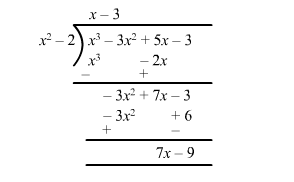# Find the quotient and the remainder when

Question:

Find the quotient and the remainder when

$f(x)=x^{3}-3 x^{2}+5 x-3$ is divided by $g(x)=x^{2}-2$

Solution:Quotient $q(x)=x-3$

Remainder $r(x)=7 x-9$